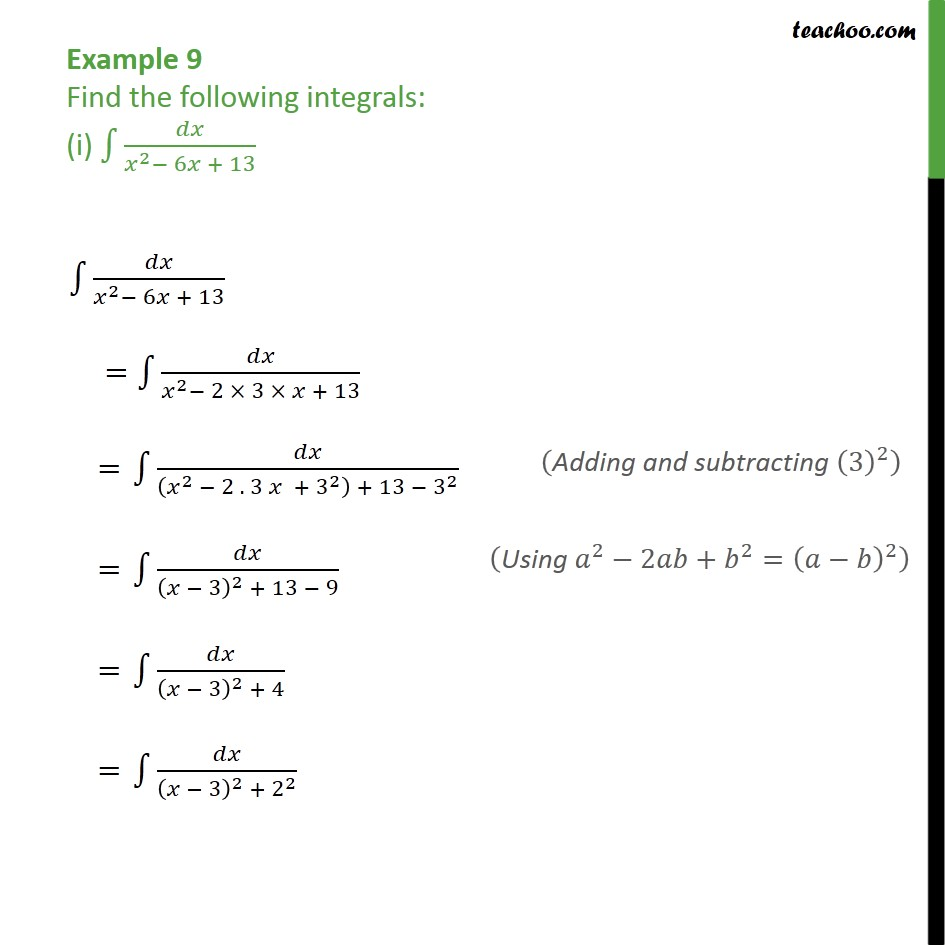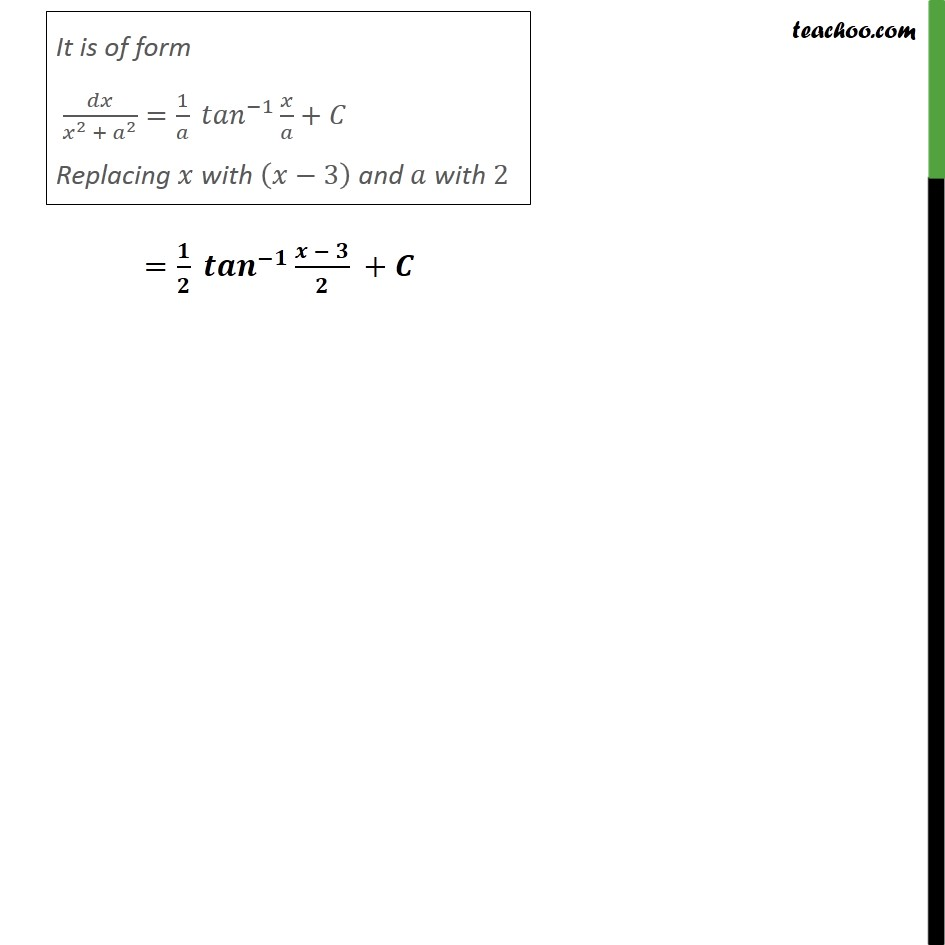Integration by specific formulaes - Formula 3

Chapter 7 Class 12 Integrals
Concept wiseLearn in your speed, with individual attention - Teachoo Maths 1-on-1 Class

### Transcript

Example 9 Find the following integrals: (i) ∫1▒𝑑𝑥/(𝑥^2− 6𝑥 + 13) ∫1▒𝑑𝑥/(𝑥^2− 6𝑥 + 13) =∫1▒𝑑𝑥/(𝑥^2− 2 × 3 × 𝑥 + 13) = ∫1▒𝑑𝑥/((𝑥^2 − 2 . 3 𝑥 + 3^2 ) + 13 − 3^2 )("Adding and subtracting " (3)^2 ) = ∫1▒𝑑𝑥/((𝑥 − 3)^2 + 13 − 9)("Using " 𝑎^2−2𝑎𝑏+𝑏^2=(𝑎−𝑏)^2 ) = ∫1▒𝑑𝑥/((𝑥 − 3)^2 + 4) = ∫1▒𝑑𝑥/((𝑥 − 3)^2 + 2^2 ) It is of form 𝑑𝑥/(𝑥^2 + 𝑎^2 )=1/𝑎 〖𝑡𝑎𝑛〗^(−1)⁡〖𝑥/𝑎〗+𝐶 Replacing 𝑥 with (𝑥−3) and 𝑎 with 2 =𝟏/𝟐 〖𝒕𝒂𝒏〗^(−𝟏)⁡〖(𝒙 − 𝟑)/𝟐〗 +𝑪